﻿ Spice up your website with machine learning!Evelina Gabasova

@evelgab

# F# Snippets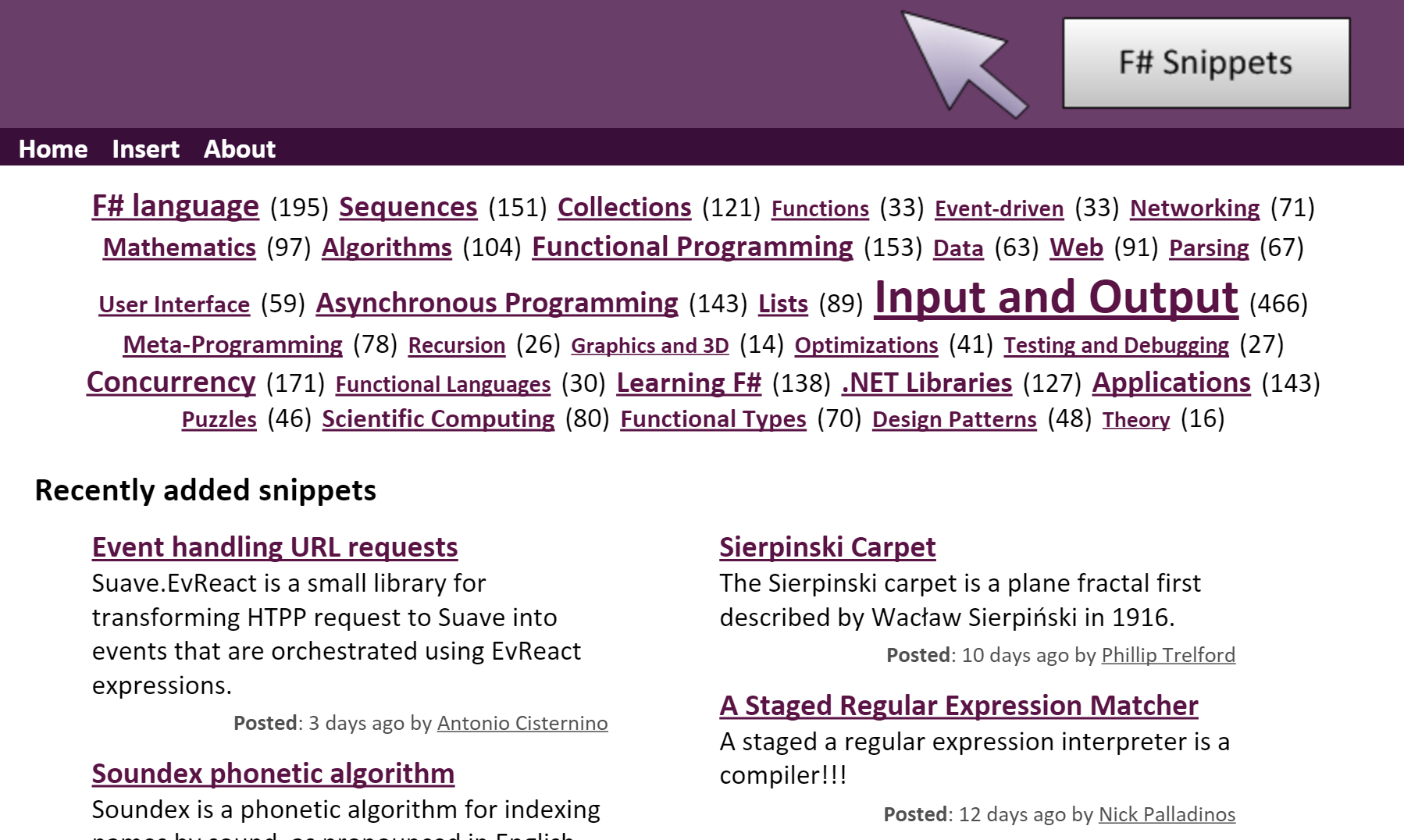fssnip.net

# Searching through F# snippets

over 1600 snippets

over 1100 different tags

# Searching through F# snippets# Do we need a custom system?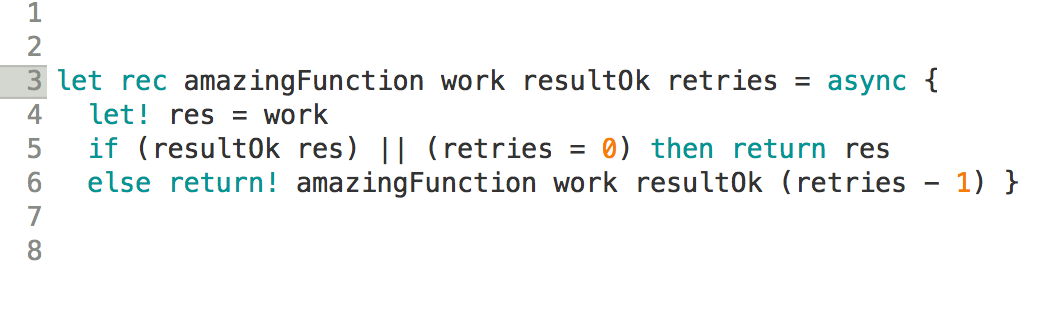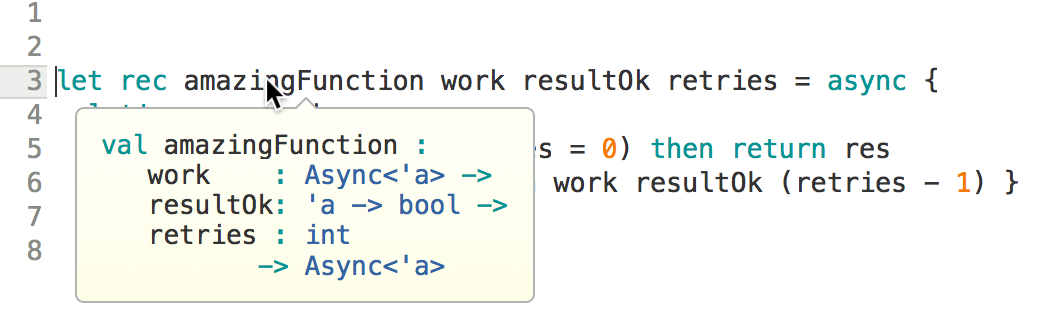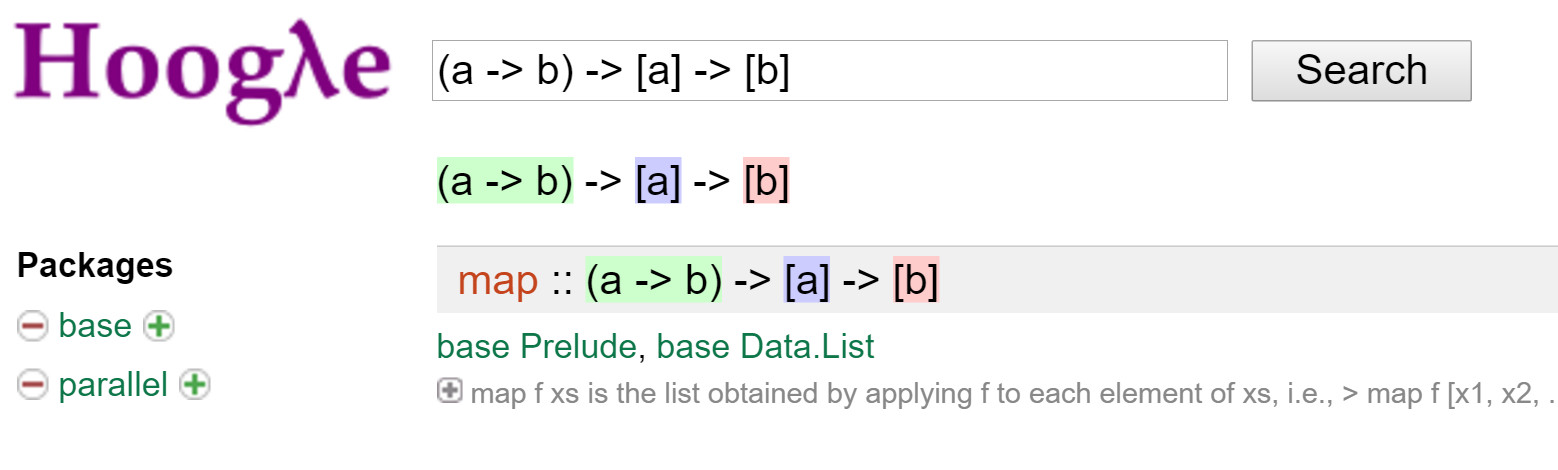## Great opportunity to create a custom machine learning system!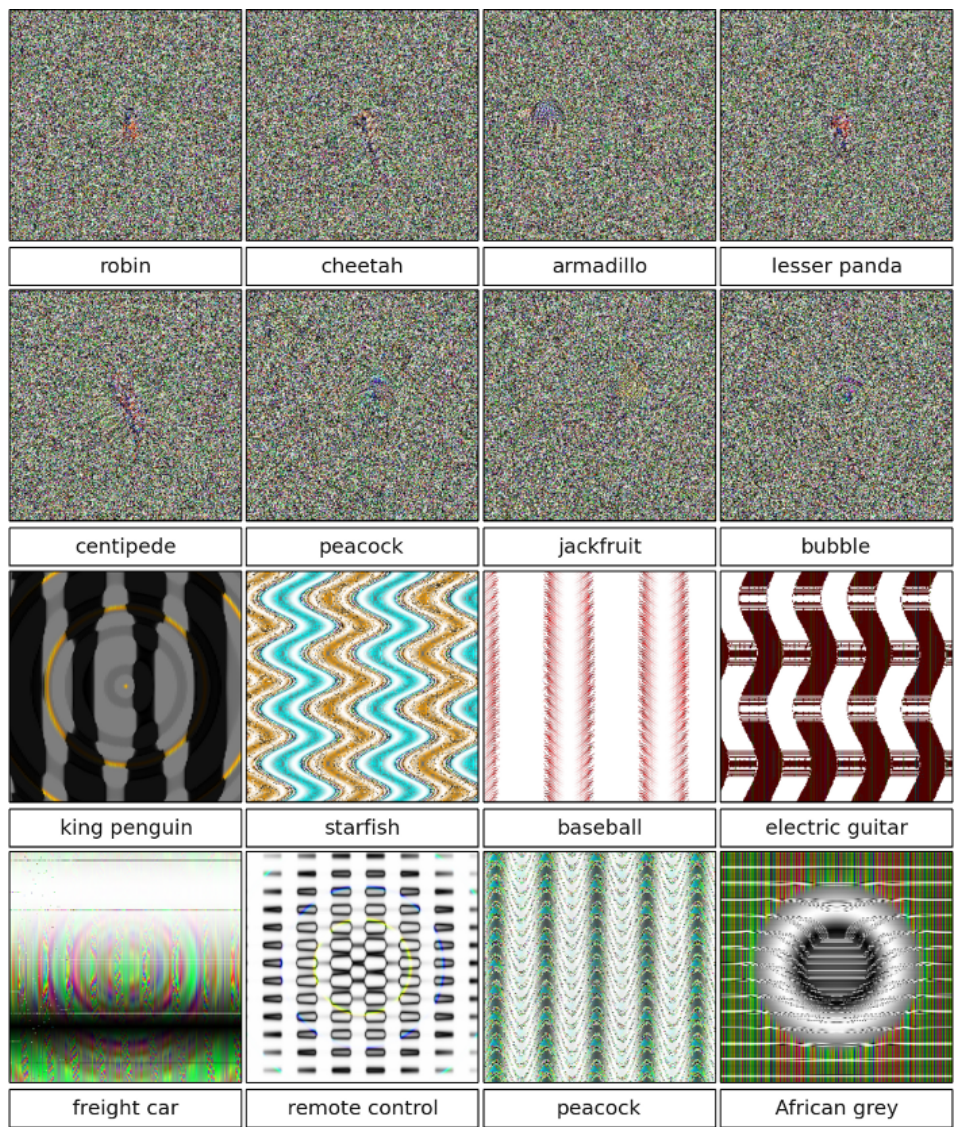Nguyen A et al.: Deep Neural Networks are Easily Fooled: High Confidence Predictions for Unrecognizable Images. 2015.

# Using machine learning in production

• dependence on training data
• inputs

# User-generated inputs• data-background : #87c594# PART I

## Finding related snippets

If you liked this F# code, you'll also like ...

common terms

# Bag of words

• ignore order of words
• separate text and code

Term

Frequency

async

3

x

15

The

2

code

1

...

Term

Frequency

async

0

x

15

The

2

code

1

...

# Inverse document frequency

## Relative importance of terms

$idf(\text{term}) = \log \frac{\text{number of snippets}}{\text{number of snippets with term}}$

# Vector representation: TF-IDF

## Term frequency - inverse document frequency

$tfidf(\text{term}, \text{snippet}) = tf(\text{term}, \text{snippet}) \times idf(\text{term})$

Snippet

x

List

Array

...

snippet1

0

0.17

0

...

snippet2

0

0.04

0.001

...

snippet3

0.23

0.005

0.31

...

snippet4

0

0

0

...

...

# Vector representation of snippets# PART II

## Suggesting tags# Suggesting tags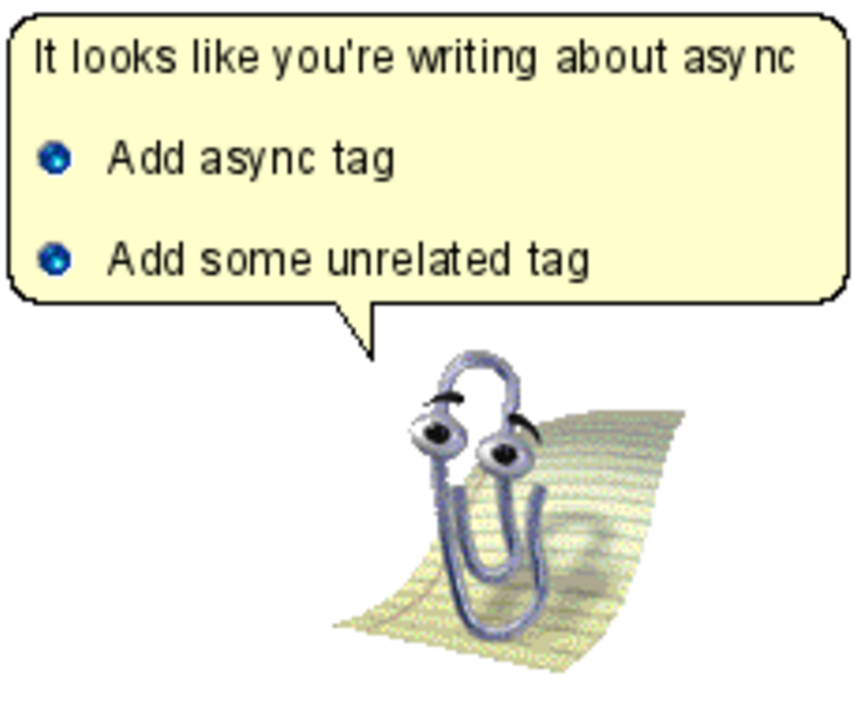# Making sense of user-generated tags

async, #async, async mailprocessor, async paraller, Async sequences, asyncseq, asynchronous, Asynchronous Processing, Asynchronous Programming, asynchronous sequence, asynchronous workflows

regex vs. regexp

sports vs. ports
pi vs. API

# Associations

string and parser

async and MailboxProcessor

sequence and exception

# Naive Bayes# Why naive?

string and parser

async and MailboxProcessor

sequence and exception

# Building a predictor# Building a predictor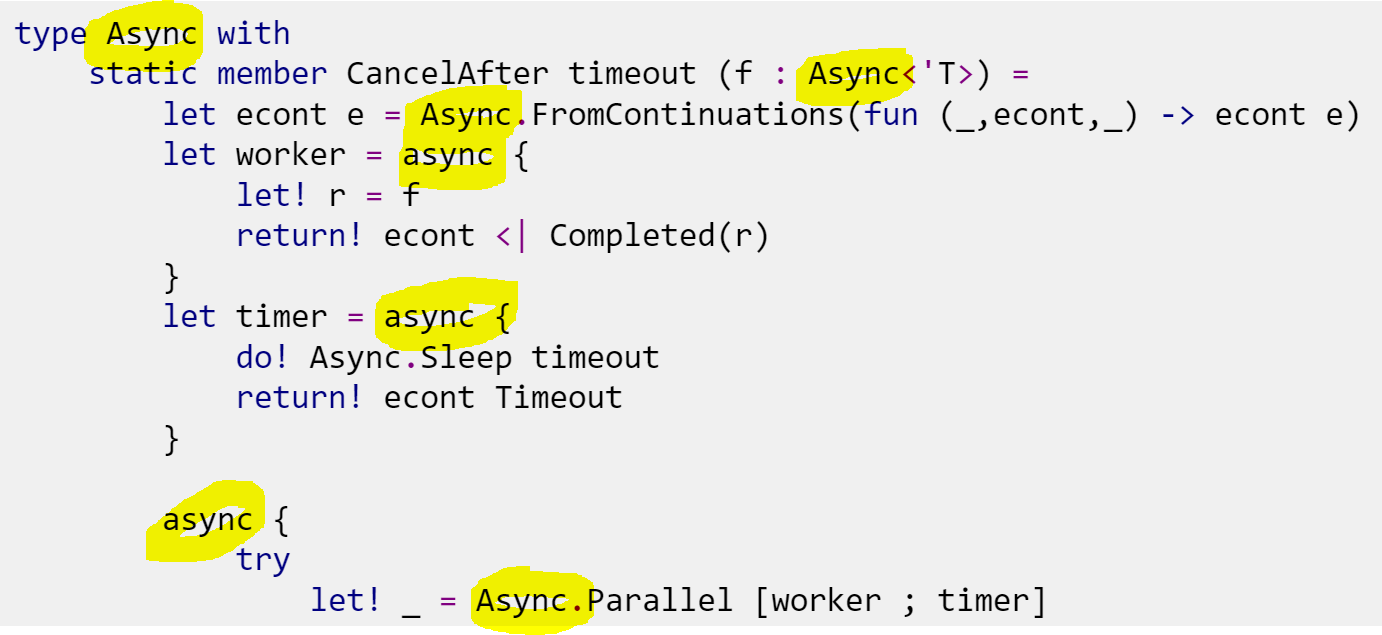# Building a predictor# Tag probabilities

## Bayes theorem

$p(A \mid B) = \frac{ p(B \mid A) \; p(A)}{p(B)}$

# Tag probabilities

## Bayes theorem

$p(\text{tag} \mid \text{snippet}) \propto p(\text{tag}) \; p(\text{snippet} \mid \text{tag} )$

# Tag probabilities

## Bayes theorem

$p(\text{tag} \mid \text{snippet}) \propto p(\text{tag}) \prod_{\text{term}} p(\text{term} \mid \text{tag})$

# Tag probabilities

## Bayes theorem

$\begin{multline*} p(\text{tag} \mid \text{snippet}) \propto p(\text{tag}) \times \\ p(\text{term}_1 \mid \text{tag}) \, p(\text{term}_2 \mid \text{tag}) \, p(\text{term}_2 \mid \text{tag}) \dots \end{multline*}$

# 1. Prior probabilities

$p(\text{tag}) \approx \frac{\text{Number of snippets with the tag}}{\text{Number of snippets}}$

# 2. Tag likelihood

How frequent is the term among snippets that have the tag ?

$p(\text{term} \mid \text{tag}) = \frac{\text{Number of snippets with the term and tag}}{\text{Number of snippets with the tag}}$

# Naive Bayes prediction

$p(\text{tag} \mid \text{snippet}) \propto p(\text{tag}) \prod_{\text{term}} p(\text{term} \mid \text{tag})$

$p(\text{tag} \mid \text{snippet}) \stackrel{?}{>} p(\neg\text{tag} \mid \text{snippet})$

# The theory is always nicer

What if there is no snippet tagged async that contains List?

# Machine learning

1. Why do you need machine learning?
3. Feature engineering.
4. Actual machine learning.
5. Profit!

# Machine learning

1. Why do you need machine learning?
3. Feature engineering.
4. Actual machine learning.
5. ...
6. Put it into production.
7. Profit!

# Machine learning

1. Why do you need machine learning?
3. Feature engineering.
4. Actual machine learning.
5. ...
6. Put it into production.
7. Profit!

# Machine learning

1. Why do you need machine learning?
3. Feature engineering.

4. Actual machine learning.
5. ...
6. Put it into production.
7. Profit!
• Do you really need a custom system?
• Domain representation
• What are important features
• Machine learning is fun!

# Thank you!@evelgabgithub.com/evelinag evelinag.com